Home » Show Running total in SSRS

# Show Running total in SSRSThis article demonstrate how to display running total or cumulative total in SSRS.

Lets understand what is cumulative total, basically a cumulative total is a sequence of partial sum of values in any given table.

It just add up the values as you go, so for any current row the cumulative total would be the total sum of all previous value including current row value as you can see in below screenshots.Assume that, if  you want to get a running total for column Value, then it would be look like as shown below.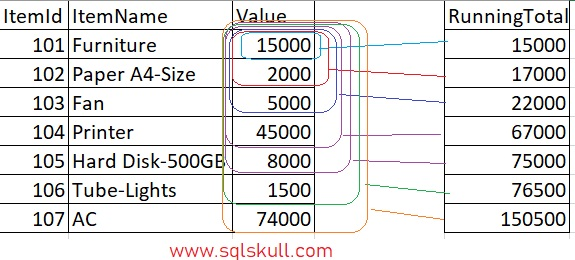You can see, for first row the cumulative total would be the sum of all previous, as there is no previous row so it would be zero 0, plus including current row value that is 15000 so the cumulative total would be 0+15000 =15000.

For second row, the cumulative total would be total sum of all previous values that is 15000, plus including current row value that is 2000 so the cumulative total for second row would be 15000+2000 =17000.

For third row, the cumulative total would be the total sum of all previous values that is 15000, 2000, plus including current row value that is 5000 so the cumulative total for third row would be 15000+2000+5000 = 22000. Similarly, it would go same like for all remaining rows.

Lets implement the cumulative total in SSRS step by step.

As you can see below, here we have a sample report. For this report we are using same dataset as you have seen above.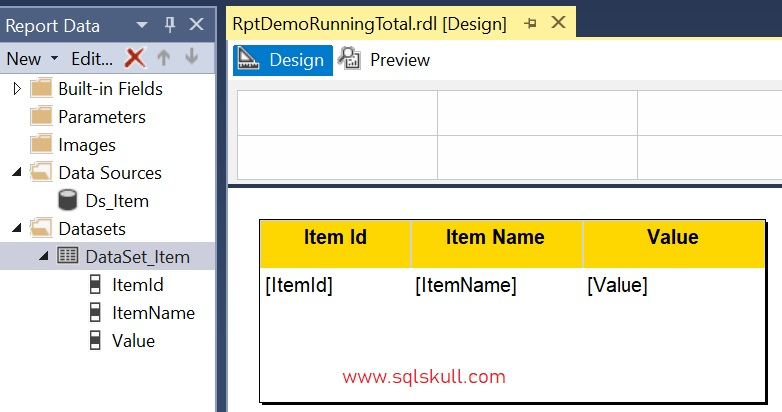Lets see the preview of report.### Displaying cumulative/running total

To display a cumulative total for column value, first we will add a new column.

Just click on last column header, and then navigate to Insert Column and select Right from context menu.Once you new column is created, just right click on this newly created column and select fxExpression… a expression window opens.

Now in Category section, expand Common functions category then click on Aggregate.

You will see the list of all aggregate function in Item section, then double click on RunningValue function.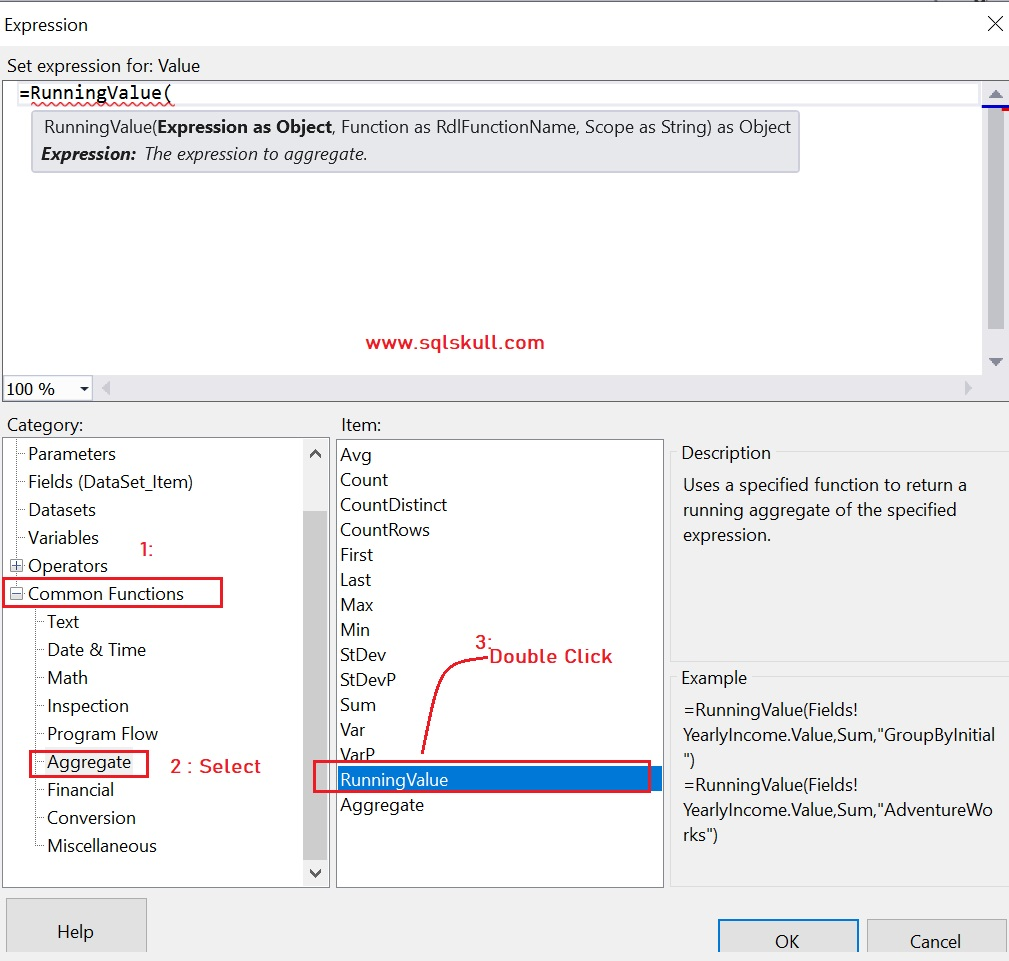After that, you will see a function expression in expression builder that we need to complete.

Now go to Fields, and double click on Value, as we want to display a running total for Value field.

After that complete the expression as shown below.

`=RunningValue(Fields!Value.Value, SUM, "DataSet_Item")`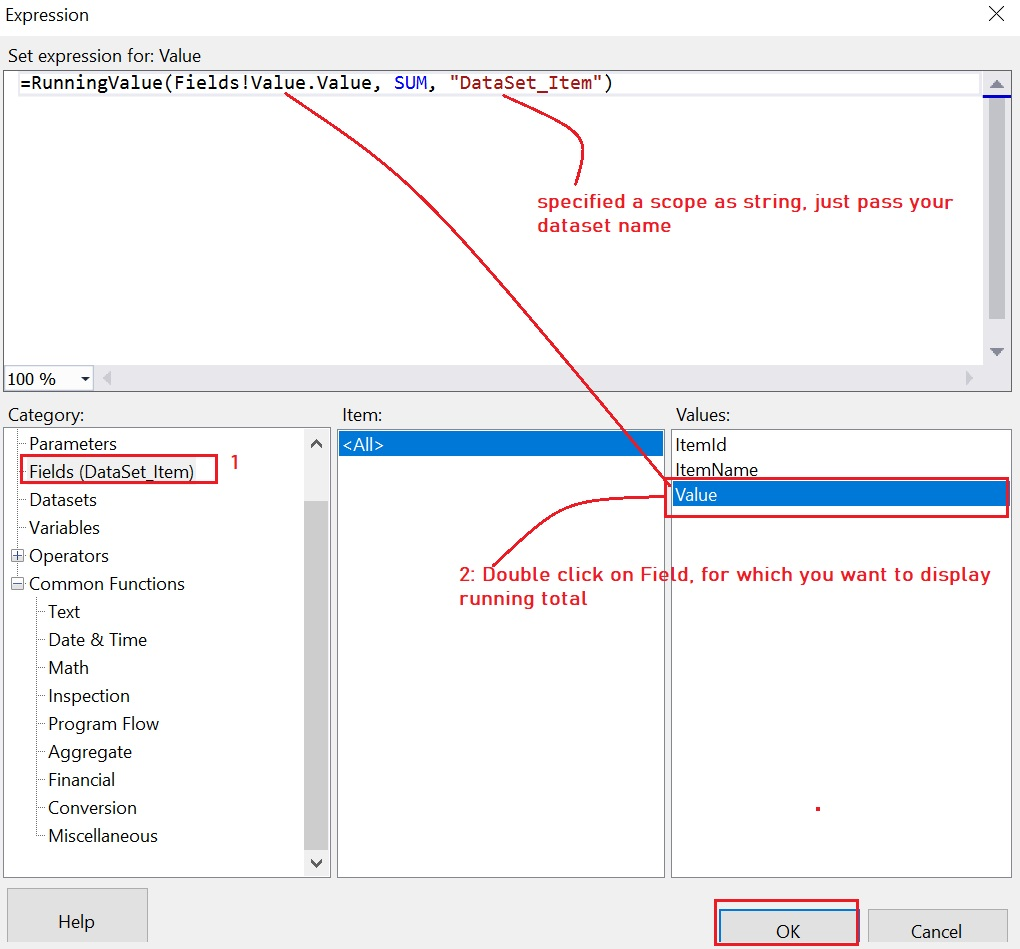Once you done with this, just click on OK button.Lets go to the preview page to see the output of report, and you can see it returns the cumulative total for Value.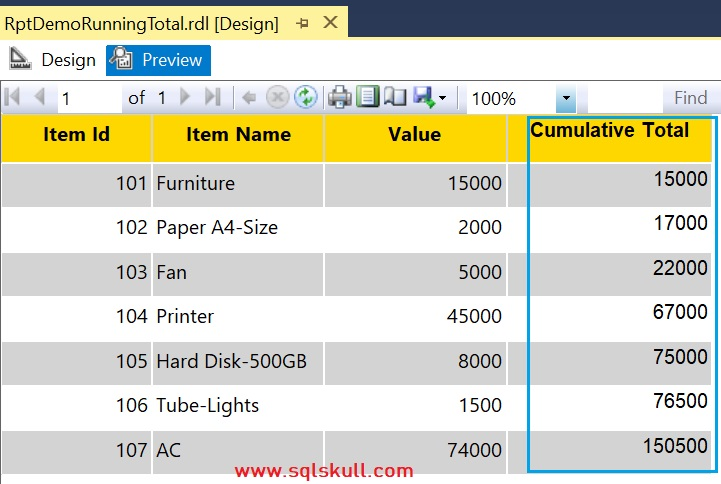Create a New SSRS Project

Create a Shared DataSource in SSRS

Create a Shared DataSet in SSRS

Create a SSRS Tabular Report / Detail Report

Create a SSRS Matrix Report

Create a List Report

Keep row header visible while scrolling down, or on each page

Parameters in SSRS

Multi – value Parameters in SSRS

SSRS Add Row Number in Report

Display checkbox for active and inactive values in SSRS report

Show “No Data Found” message when no rows returns in SSRS

817 total views,  4 views today

This site uses Akismet to reduce spam. Learn how your comment data is processed.